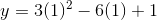# High School Math : Solving Parabola Functions

## Example Questions

### Example Question #3 : Pre Calculus

Find the vertex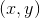for a parabola with equation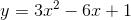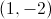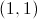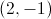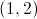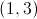Explanation:

For any parabola of the form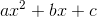,  the-coordinate of its vertex is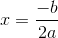So here, we have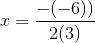We plug this back into the original equation to find: